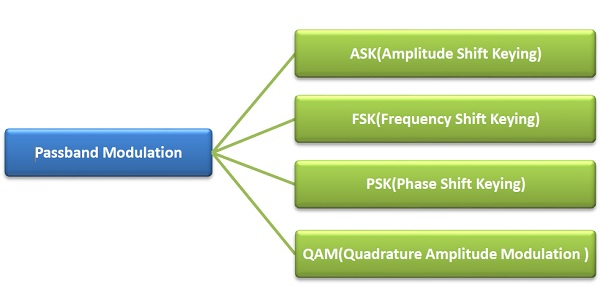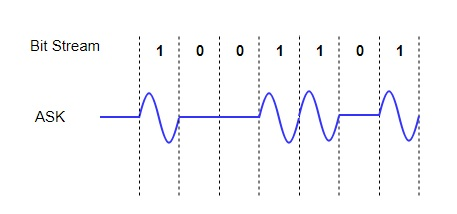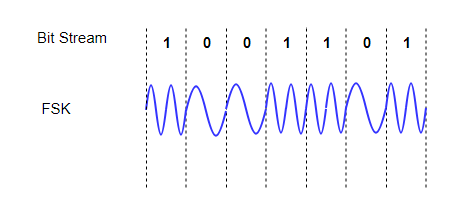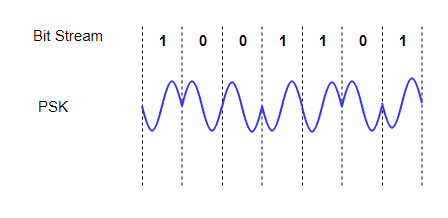# Passband Transmission

Computer NetworkComputer EngineeringMCA

#### Artificial Neural Network and Machine Learning using MATLAB

54 Lectures 4 hours

In passband transmission, the amplitude, phase or frequency of the carrier signal is regulated to transmit the bits. The incoming data stream is modulated onto a carrier and then transmitted over a band-pass channel.

The types of passband transmission are illustrated as −## Amplitude Shift Keying (ASK)

In ASK, the amplitude of the signal is varied to represent the signal levels, while frequency and phase remains constant. In order to represent 0 and 1, two different amplitudes are used.## Frequency Shift Keying (FSK)

In FSK, the frequency of the signal is modulated to represent the signal levels, while amplitude and phase remains constant. To represent the signal levels 0 and 1, two different frequencies are used.## Phase Shift Keying (PSK)

In PSK, the phase of the carrier signal is modulated to represent the signal levels, while amplitude and frequency remains constant. Binary Phase Shift Keying (BPSK) is the simplest form of PSK where there are two signal elements represented by two different phases. In Quadrature PSK (QPSK), two bits of information are transmitted per symbol by using four different phases.## Quadrature Amplitude Modulation (QAM)

QAM is a combination of ASK and PSK. Here, both the amplitude and the phase are are varied to transmit more bits per symbol.